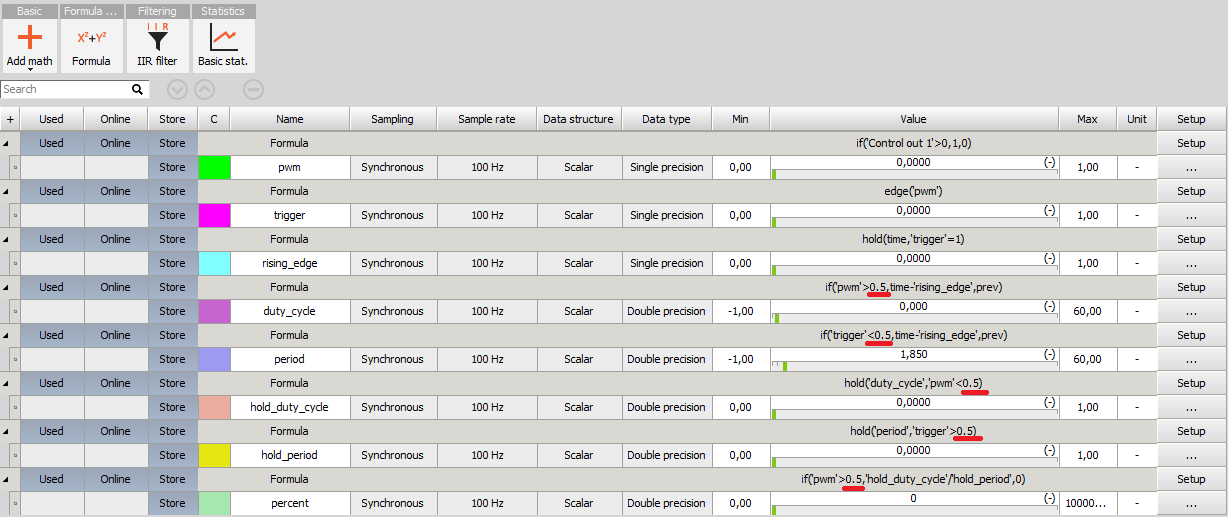# Measure Raw PWM Voltage Then Calculate % Duty Cycle?

• Software
• Measure Raw PWM Voltage Then Calculate % Duty Cycle?
Matt Hanks

Posted on 06.11.2020 05:34

Hello,

I'm reading a raw PWM voltage signal using an analog input. Is there a way in Math to calculate the % duty cycle?

Matic Pevec
Customer Support Engineer
Posted on 10.11.2020 17:44

Dear Matt,

Please find attached example setup in which I use a few Math channels to calculate the percent of PWM signal. Creating the PWM signal changes manually with push button. Attached is also video how it works.

"trigger" formula is used to set the trigger signal where PWM pulse starts.

"rising_edge" formula remembers the time where PWM pulse starts.

"duty_cycle" and "period" formulas calculates elapsed time from rising edge of the PWM

"hold_duty_cycle" and "hold_period" holds values for calculating the percent of PWM.

"percent" channel only shows value when he PWM is one.

Please note that you should change the marked values according to the characteristics of your signaKind regards

Attached files:
Login to reply to this topic. If you don't have account yet, you can signup for free account .